# Selina Solutions Concise Maths Class 10 Chapter 10 Arithmetic Progression Exercise 10(A)

Basic terminologies of A.P and its general term calculation are discussed in this exercise. Problems pertaining to these concepts are practised under the exercise 10(A). Students having any doubts regarding solving problems of this exercise can refer to Selina Solutions Concise Maths Class 10 Chapter 10 Exercise 10(A). Further, the answers to this exercise can be accessed in the Selina Solutions Concise Maths for Class 10 Chapter 10 Arithmetic Progression Exercise 10(A) PDF, which is given in the links below.

## Selina Solutions Concise Maths Class 10 Chapter 10 Arithmetic Progression Exercise 10(A) Download PDF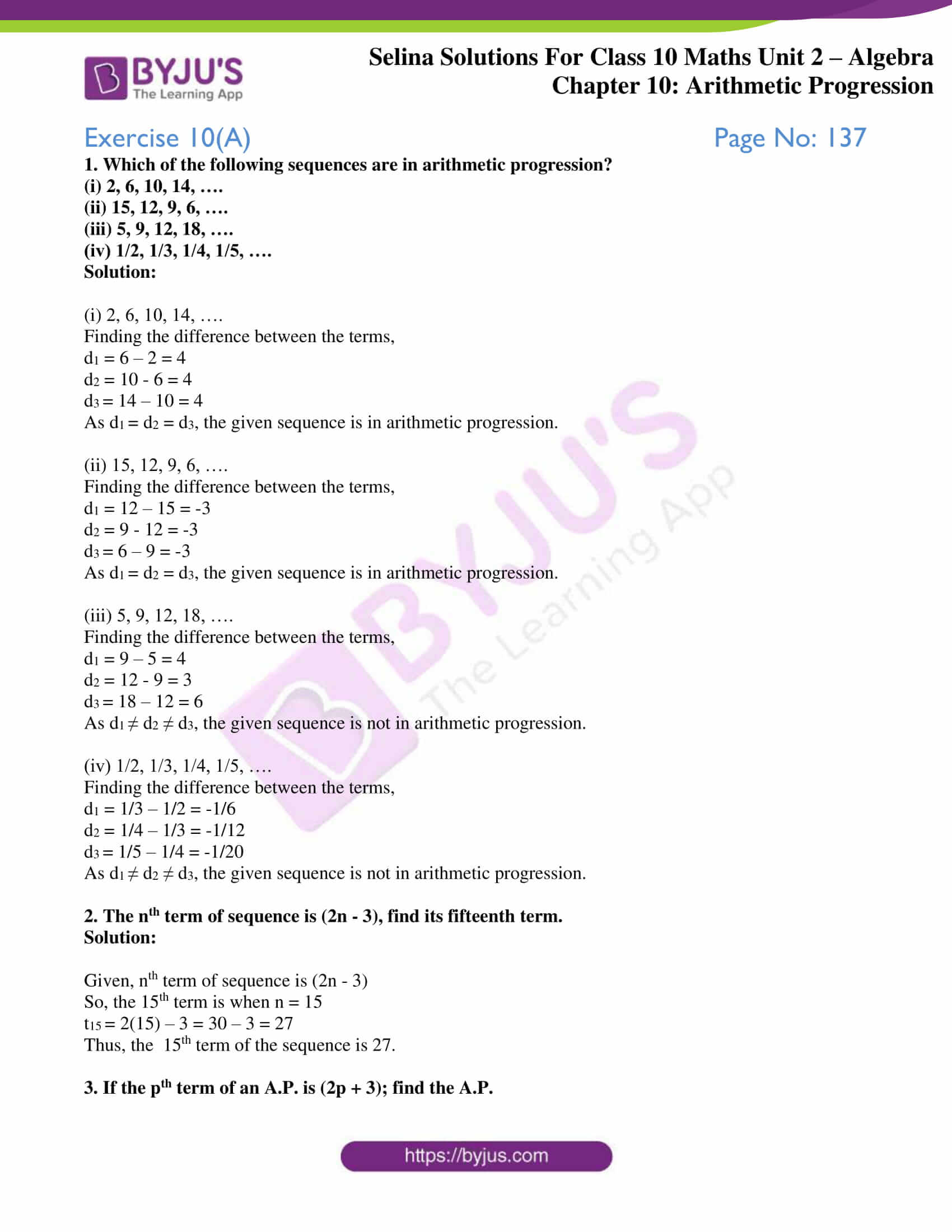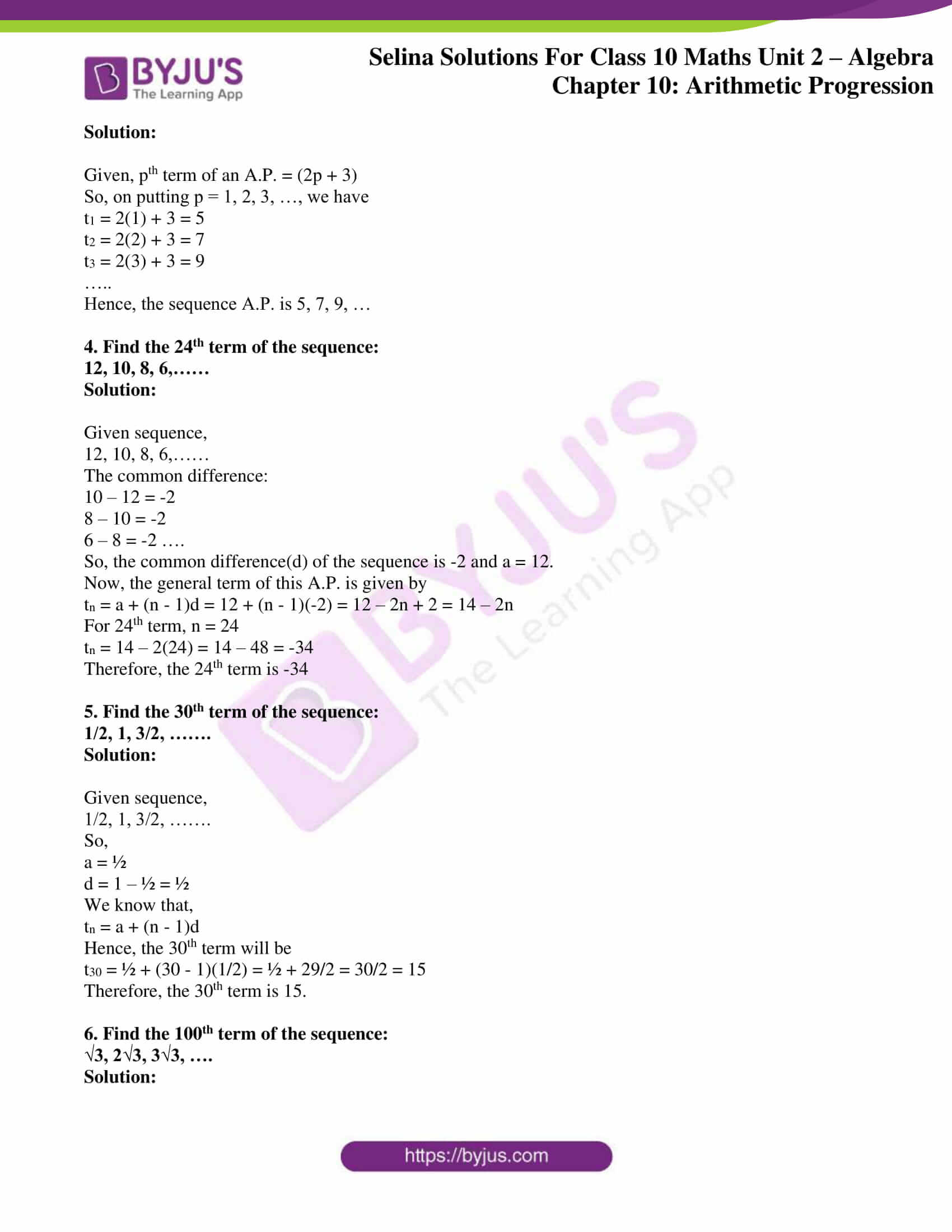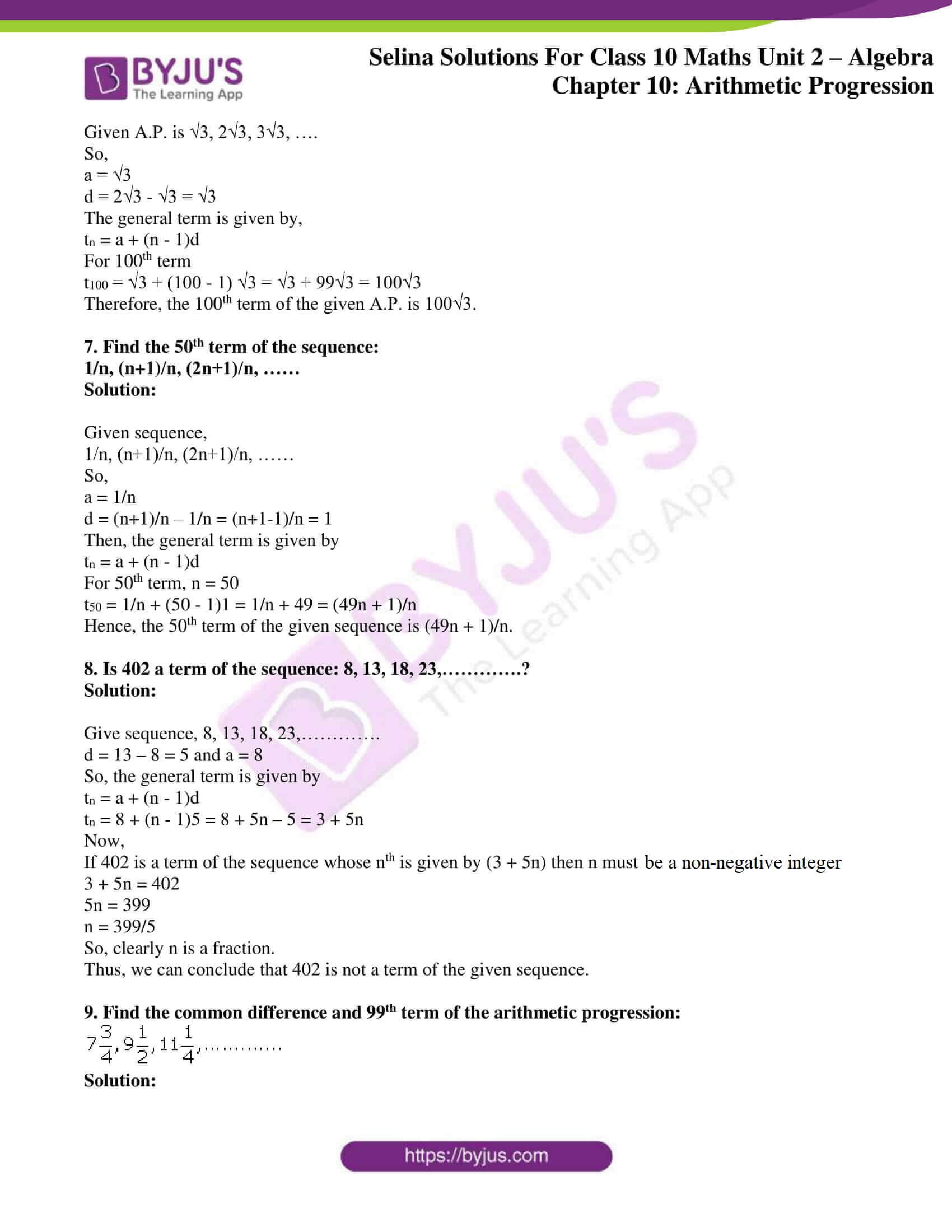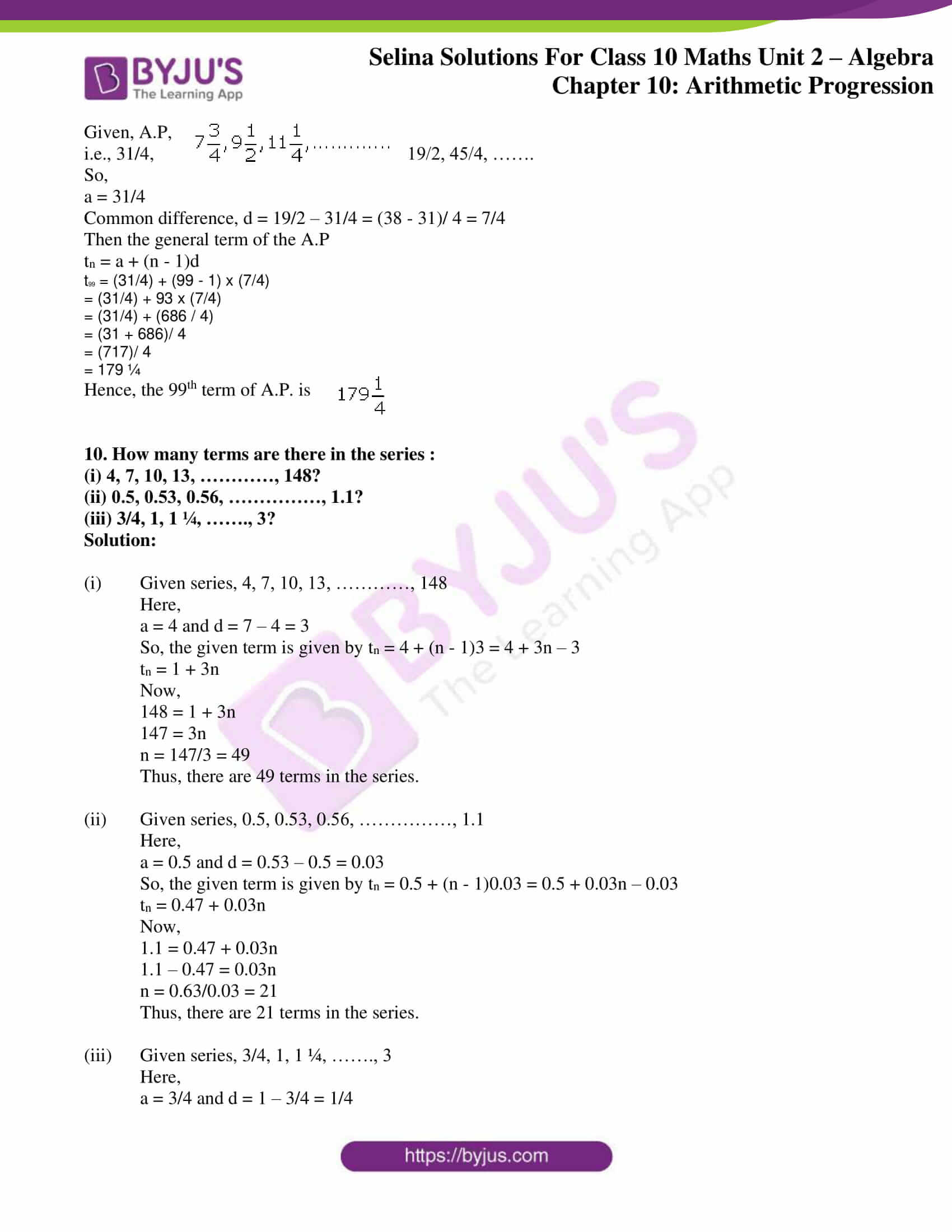### Access Selina Solutions Concise Maths Class 10 Chapter 10 Arithmetic Progression Exercise 10(A)

#### Exercise 10(A) Page No: 137

1. Which of the following sequences are in arithmetic progression?

(i) 2, 6, 10, 14, ….

(ii) 15, 12, 9, 6, ….

(iii) 5, 9, 12, 18, ….

(iv) 1/2, 1/3, 1/4, 1/5, ….

Solution:

(i) 2, 6, 10, 14, ….

Finding the difference between the terms,

d1 = 6 – 2 = 4

d2 = 10 – 6 = 4

d3 = 14 – 10 = 4

As d1 = d2 = d3, the given sequence is in arithmetic progression.

(ii) 15, 12, 9, 6, ….

Finding the difference between the terms,

d1 = 12 – 15 = -3

d2 = 9 – 12 = -3

d3 = 6 – 9 = -3

As d1 = d2 = d3, the given sequence is in arithmetic progression.

(iii) 5, 9, 12, 18, ….

Finding the difference between the terms,

d1 = 9 – 5 = 4

d2 = 12 – 9 = 3

d3 = 18 – 12 = 6

As d1 ≠ d2 ≠ d3, the given sequence is not in arithmetic progression.

(iv) 1/2, 1/3, 1/4, 1/5, ….

Finding the difference between the terms,

d1 = 1/3 – 1/2 = -1/6

d2 = 1/4 – 1/3 = -1/12

d3 = 1/5 – 1/4 = -1/20

As d1 ≠ d2 ≠ d3, the given sequence is not in arithmetic progression.

2. The nth term of sequence is (2n – 3), find its fifteenth term.

Solution:

Given, nth term of sequence is (2n – 3)

So, the 15th term is when n = 15

t15 = 2(15) – 3 = 30 – 3 = 27

Thus, the 15th term of the sequence is 27.

3. If the pth term of an A.P. is (2p + 3); find the A.P.

Solution:

Given, pth term of an A.P. = (2p + 3)

So, on putting p = 1, 2, 3, …, we have

t1 = 2(1) + 3 = 5

t2 = 2(2) + 3 = 7

t3 = 2(3) + 3 = 9

…..

Hence, the sequence A.P. is 5, 7, 9, …

4. Find the 24th term of the sequence:

12, 10, 8, 6,……

Solution:

Given sequence,

12, 10, 8, 6,……

The common difference:

10 – 12 = -2

8 – 10 = -2

6 – 8 = -2 ….

So, the common difference(d) of the sequence is -2 and a = 12.

Now, the general term of this A.P. is given by

tn = a + (n – 1)d = 12 + (n – 1)(-2) = 12 – 2n + 2 = 14 – 2n

For 24th term, n = 24

tn = 14 – 2(24) = 14 – 48 = -34

Therefore, the 24th term is -34

5. Find the 30th term of the sequence:

1/2, 1, 3/2, …….

Solution:

Given sequence,

1/2, 1, 3/2, …….

So,

a = ½

d = 1 – ½ = ½

We know that,

tn = a + (n – 1)d

Hence, the 30th term will be

t30 = ½ + (30 – 1)(1/2) = ½ + 29/2 = 30/2 = 15

Therefore, the 30th term is 15.

6. Find the 100th term of the sequence:

√3, 2√3, 3√3, ….

Solution:

Given A.P. is √3, 2√3, 3√3, ….

So,

a = √3

d = 2√3 – √3 = √3

The general term is given by,

tn = a + (n – 1)d

For 100th term

t100 = √3 + (100 – 1) √3 = √3 + 99√3 = 100√3

Therefore, the 100th term of the given A.P. is 100√3.

7. Find the 50th term of the sequence:

1/n, (n+1)/n, (2n+1)/n, ……

Solution:

Given sequence,

1/n, (n+1)/n, (2n+1)/n, ……

So,

a = 1/n

d = (n+1)/n – 1/n = (n+1-1)/n = 1

Then, the general term is given by

tn = a + (n – 1)d

For 50th term, n = 50

t50 = 1/n + (50 – 1)1 = 1/n + 49 = (49n + 1)/n

Hence, the 50th term of the given sequence is (49n + 1)/n.

8. Is 402 a term of the sequence: 8, 13, 18, 23,………….?

Solution:

Give sequence, 8, 13, 18, 23,………….

d = 13 – 8 = 5 and a = 8

So, the general term is given by

tn = a + (n – 1)d

tn = 8 + (n – 1)5 = 8 + 5n – 5 = 3 + 5n

Now,

If 402 is a term of the sequence whose nth is given by (3 + 5n) then n must be a non-negative integer.

3 + 5n = 402

5n = 399

n = 399/5

So, clearly n is a fraction.

Thus, we can conclude that 402 is not a term of the given sequence.

9. Find the common difference and 99th term of the arithmetic progression: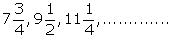Solution:Given, A.P,

i.e., 31/4, 19/2, 45/4, …….

So,

a = 31/4

Common difference, d = 19/2 – 31/4 = (38 – 31)/ 4 = 7/4

Then the general term of the A.P

tn = a + (n – 1)d

t99 = (31/4) + (99 – 1) x (7/4)

= (31/4) + 93 x (7/4)

= (31/4) + (686 / 4)

= (31 + 686)/ 4

= (717)/ 4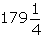= 179 ¼

Hence, the 99th term of A.P. is

10. How many terms are there in the series :

(i) 4, 7, 10, 13, …………, 148?

(ii) 0.5, 0.53, 0.56, ……………, 1.1?

(iii) 3/4, 1, 1 ¼, ……., 3?

Solution:

(i) Given series, 4, 7, 10, 13, …………, 148

Here,

a = 4 and d = 7 – 4 = 3

So, the given term is given by tn = 4 + (n – 1)3 = 4 + 3n – 3

tn = 1 + 3n

Now,

148 = 1 + 3n

147 = 3n

n = 147/3 = 49

Thus, there are 49 terms in the series.

(ii) Given series, 0.5, 0.53, 0.56, ……………, 1.1

Here,

a = 0.5 and d = 0.53 – 0.5 = 0.03

So, the given term is given by tn = 0.5 + (n – 1)0.03 = 0.5 + 0.03n – 0.03

tn = 0.47 + 0.03n

Now,

1.1 = 0.47 + 0.03n

1.1 – 0.47 = 0.03n

n = 0.63/0.03 = 21

Thus, there are 21 terms in the series.

(iii) Given series, 3/4, 1, 1 ¼, ……., 3

Here,

a = 3/4 and d = 1 – 3/4 = 1/4

So, the given term is given by tn = 3/4 + (n – 1)1/4 = (3 + n – 1)/4

tn = (2 + n)/ 4

Now,

3 = (2 + n)/ 4

12 = 2 + n

n = 10

Thus, there are 10 terms in the series.

### Access other exercises of Selina Solutions Concise Maths Class 10 Chapter 10 Arithmetic Progression

Exercise 10(B) Solutions

Exercise 10(C) Solutions

Exercise 10(D) Solutions

Exercise 10(E) Solutions

Exercise 10(F) Solutions